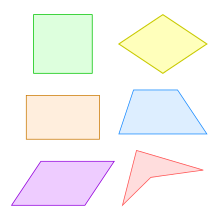3 years ago
thertenstein
Save
Edit
Host a game
Live GameLive
Homework
Solo Practice
Practice10 QuestionsShow answers
• Question 1
120 secondsQ. Name that shape.
square
square
rhombus
parallelogram
rhombus
parallelogram
square
• Question 2
120 secondsQ. Name that shape.
trapezoid
parallelogram
rhombus
• Question 3
120 secondsQ. Name that shape.
• Question 4
120 seconds
Q. A __________________ is always a rhombus, but a rhombus is not always a _______________.
kite
parallelogram
square
rectangle
• Question 5
120 seconds
Q. If a quadrilateral has 4 equal sides, then it is a square.
TRUE
FALSE
• Question 6
60 seconds
Q. A trapezoid is a quadrilateral with exactly ONE set of parallel sides.
TRUE
FALSE
• Question 7
120 secondsQ. A __________________ is a quadrilateral with two sets of parallel sides and 4 right angles.
trapezoid
parallelogram
rectangle
square
• Question 8
30 seconds
Q. A quadrilateral with two sets of parallel sides, 4 right angles, and 4 congruent (equal) sides is a __________________.
trapezoid
parallelogram
rectangle
square
• Question 9
120 seconds
Q. A ___________ is a quadrilateral with two sets or parallel sides and 4 congruent (equal) sides.
trapezoid
rhombus
parallelogram
rectangle
• Question 10
120 seconds
Q. A _______________ is a quadrilateral with two sets of parallel sides.